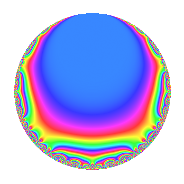# Properties

 Label 731.2.d.aLevel 731 Weight 2 Character orbit 731.d Analytic conductor 5.837 Analytic rank 0 Dimension 2 CM No Inner twists 2

# Related objects

## Newspace parameters

 Level: $$N$$ = $$731 = 17 \cdot 43$$ Weight: $$k$$ = $$2$$ Character orbit: $$[\chi]$$ = 731.d (of order $$2$$ and degree $$1$$)

## Newform invariants

 Self dual: No Analytic conductor: $$5.83706438776$$ Analytic rank: $$0$$ Dimension: $$2$$ Coefficient field: $$\Q(\sqrt{-2})$$ Coefficient ring: $$\Z[a_1, \ldots, a_{5}]$$ Coefficient ring index: $$2$$ Sato-Tate group: $\mathrm{SU}(2)[C_{2}]$

## $q$-expansion

Coefficients of the $$q$$-expansion are expressed in terms of $$\beta = 2\sqrt{-2}$$. We also show the integral $$q$$-expansion of the trace form.

 $$f(q)$$ $$=$$ $$q + q^{2} - q^{4} + \beta q^{5} -3 q^{8} + 3 q^{9} +O(q^{10})$$ $$q + q^{2} - q^{4} + \beta q^{5} -3 q^{8} + 3 q^{9} + \beta q^{10} + \beta q^{11} -6 q^{13} - q^{16} + ( -3 - \beta ) q^{17} + 3 q^{18} -4 q^{19} -\beta q^{20} + \beta q^{22} + \beta q^{23} -3 q^{25} -6 q^{26} -\beta q^{29} + 3 \beta q^{31} + 5 q^{32} + ( -3 - \beta ) q^{34} -3 q^{36} + 3 \beta q^{37} -4 q^{38} -3 \beta q^{40} + 2 \beta q^{41} + q^{43} -\beta q^{44} + 3 \beta q^{45} + \beta q^{46} + 7 q^{49} -3 q^{50} + 6 q^{52} -10 q^{53} -8 q^{55} -\beta q^{58} -4 q^{59} -3 \beta q^{61} + 3 \beta q^{62} + 7 q^{64} -6 \beta q^{65} + 12 q^{67} + ( 3 + \beta ) q^{68} -4 \beta q^{71} -9 q^{72} + 5 \beta q^{73} + 3 \beta q^{74} + 4 q^{76} -3 \beta q^{79} -\beta q^{80} + 9 q^{81} + 2 \beta q^{82} + 4 q^{83} + ( 8 - 3 \beta ) q^{85} + q^{86} -3 \beta q^{88} + 6 q^{89} + 3 \beta q^{90} -\beta q^{92} -4 \beta q^{95} + 4 \beta q^{97} + 7 q^{98} + 3 \beta q^{99} +O(q^{100})$$ $$\operatorname{Tr}(f)(q)$$ $$=$$ $$2q + 2q^{2} - 2q^{4} - 6q^{8} + 6q^{9} + O(q^{10})$$ $$2q + 2q^{2} - 2q^{4} - 6q^{8} + 6q^{9} - 12q^{13} - 2q^{16} - 6q^{17} + 6q^{18} - 8q^{19} - 6q^{25} - 12q^{26} + 10q^{32} - 6q^{34} - 6q^{36} - 8q^{38} + 2q^{43} + 14q^{49} - 6q^{50} + 12q^{52} - 20q^{53} - 16q^{55} - 8q^{59} + 14q^{64} + 24q^{67} + 6q^{68} - 18q^{72} + 8q^{76} + 18q^{81} + 8q^{83} + 16q^{85} + 2q^{86} + 12q^{89} + 14q^{98} + O(q^{100})$$

## Character Values

We give the values of $$\chi$$ on generators for $$\left(\mathbb{Z}/731\mathbb{Z}\right)^\times$$.

 $$n$$ $$173$$ $$562$$ $$\chi(n)$$ $$-1$$ $$1$$

## Embeddings

For each embedding $$\iota_m$$ of the coefficient field, the values $$\iota_m(a_n)$$ are shown below.

For more information on an embedded modular form you can click on its label.

Label $$\iota_m(\nu)$$ $$a_{2}$$ $$a_{3}$$ $$a_{4}$$ $$a_{5}$$ $$a_{6}$$ $$a_{7}$$ $$a_{8}$$ $$a_{9}$$ $$a_{10}$$
560.1
 − 1.41421i 1.41421i
1.00000 0 −1.00000 2.82843i 0 0 −3.00000 3.00000 2.82843i
560.2 1.00000 0 −1.00000 2.82843i 0 0 −3.00000 3.00000 2.82843i
 $$n$$: e.g. 2-40 or 990-1000 Significant digits: Format: Complex embeddings Normalized embeddings Satake parameters Satake angles

## Inner twists

Char. orbit Parity Mult. Self Twist Proved
1.a Even 1 trivial yes
17.b Even 1 yes

## Hecke kernels

This newform can be constructed as the kernel of the linear operator $$T_{2} - 1$$ acting on $$S_{2}^{\mathrm{new}}(731, [\chi])$$.